Engineering Jobs   »   Civil Engineering Quiz

# RSMSSB-JE’21 CE: Weekly Practices Quiz. 05-Sep-2021

As RSMSSB JE exam date 2021 is announced which is going to be held on 12th September 2021. As RSMSSB JE exam date 2021 is nearby so boost your preparation by attempt this Civil Engineering miscellaneous quiz for RSMSSB JE recruitment 2021.

Quiz: Civil Engineering
Exam: RSMSSB-JEn
Topic: Miscellaneous

Each question carries 1 mark
Negative marking: 1/3 mark
Time: 12 Minutes

Q1. What will be the dry density of soil if a soil has a bulk density of 22 kN/m^3 and water content 10%
(a) 18.6 kN\/m^3
(b) 22.0 kN\/m^2
(c) 23.2 kN\/m^3
(d) 20.0 kN\/m^3

Q2. The process of mixing water with quick lime is called:
(a) Cooling of lime
(b) Slaking of lime
(c) Curing of lime
(d) Calcination of lime

Q3. The quantity of cement required in 1 cum of 1:2:4 cement concrete is approximately
(a) 0.11 cu m
(b) 0.22 cu m
(c) 0.33 cu m
(d) 0.44 cu m

Q4. If the reduced bearing of a line AB is N60° W and length is 100 m, then the latitude and departure respectively of the line AB will be
(a) +50 m, + 86.6 m
(b) +86.6 m, –50m
(c) +50 m, –86.6 m
(d) + 70.7 m, –50m

Q5. What shall be the pressure intensity inside a soap bubble of radius 4 cm? (Surface tension of water is 0.0736 N/m):
(a) 7.36 N/m^2
(b) 1.84 N/m^2
(c) 3.68 N/m^2
(d) None of these

Q6. Loam is
(a) A mixture of sand, silt and clay
(b) A mixture of gravel, sand and silt
(c) An impure form of limestone
(d) Is a wind blow deposit

Q7. Calculate the value of modulus of rigidity (N/mm²) if the Poisson’s ratio is 0.25 and modulus of elasticity for the material is 200 N/mm²
(a) 50
(b) 80
(c) 100
(d) 150

Q8. If the distance between two-point A and B is 1 km, the combined correction for curvature and refraction will be
(a) 0.6730 m
(b) 0.06735
(c) 1.0673
(d) 1.6730

Q9. Outer projection of ‘Tread’ is called
(a) Nosing
(c) Step
(d) Going

Q10. Clay can be described as:
(a) Aquifer
(b) Aquitard
(c) Aquiclude
(d) None of these

Q11. When the degree of consolidation is 50% about how much is the time factor?
(a) 0.5
(b) 2.0
(c) 1.0
(d) 0.2

Q12. Bolts are most suitable to carry-
(a) Shear
(b) Bending
(c) Axial tension
(d) Shear and bending

Solutions

S1. Ans.(d)
Sol. Bulk density (Υ_b )=22 kN\/m^3
Water content (w)=10%
Dry density (Υ_d )=Υ_b/(1+w)
=22/(1+0.1)
=20.0 kN\/m^3

S2. Ans.(b)
Sol. Slaked lime is obtained when quick lime reacts with water, this reaction is highly exothermic. This process is known as slaking of lime. During slaking process, the volume of lime increases.
CaO+H_2 O→Ca(OH)_2+Heat

S3. Ans.(b)
Sol. The volume of dry concrete is 1.52 times of quantity of quantity of wet concrete.
Hence.
Quantity of dry cement for 1 m³ wet cement concrete = 1 × 1.52 = 1.52 m³.
Quantity of cement in 1:2:4 proportion of cement = 1/7×1.52=0.217 m^3 ≃ 0.22 m³.

S4. Ans.(c)
Sol. Reduced bearing = N 60° W
Length = 100 m
Latitude = 100 cos 60°= 50 m
Departure = – 100 sin 60° = – 86.6 m

S5. Ans.(a)
Sol. Surface tension (σ)=0.0736 N/m
Pressure intensity inside soap bubble ⇒ 4σ/r
=(4×0.0736)/(4×10^(-2) )
=7.36 N\/m^2

S6. Ans.(a)
Sol. Loam is a mixture of sand, silt and clay.

S7. Ans.(b)
Sol. Given; E = 200 N/mm², G = ?
μ = 0.25
we know that,
E=2G(1+μ)
200=2G(1+0.25)
100=G(1.25)
G=80 N\/mm^2

S8. Ans.(b)
Sol. Combined correction due to curvature & Refraction (Cc) = 0.0673 d^2
=0.0673×1^2
=0.0673 m.

S9. Ans.(a)
Sol. Noising → It is the outer projection of a tread beyond the face of the riser. It is rounded to give good architectural view.

S10. Ans.(c)
Sol. Aquiclude are geological formation or stratum of impervious material which do not allow of water. Clay is an example of aquiclude.

S11. Ans.(d)
Sol. When %U < 60%
time factor (T_v )=π/4 (%U)^2
T_V=3.14/4 (0.50)^2
▭(T_v=0.19625)
▭(T_v≈0.2)

S12. Ans.(c)
Sol. Bolts are more suitable to carry Axial tension.

Sharing is caring!

### TOPICS:

•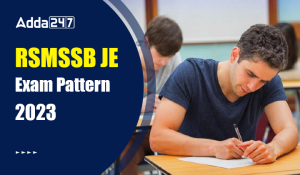RSMSSB JE Exam Pattern 2023, Check Lates...
•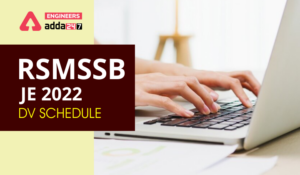RSMSSB JE 2022 DV Schedule, Download RSM...
•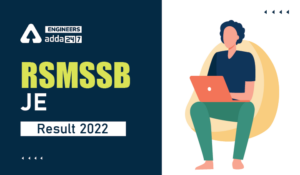RSMSSB JE Result 2022, OUT-Direct Link t...
•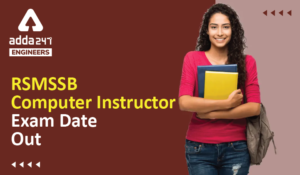RSMSSB Computer Instructor Exam date 202...
•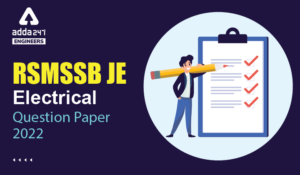RSMSSB JE Electrical Question Paper 2022...
•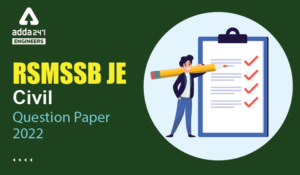RSMSSB JE Civil Question Paper 2022, Che...